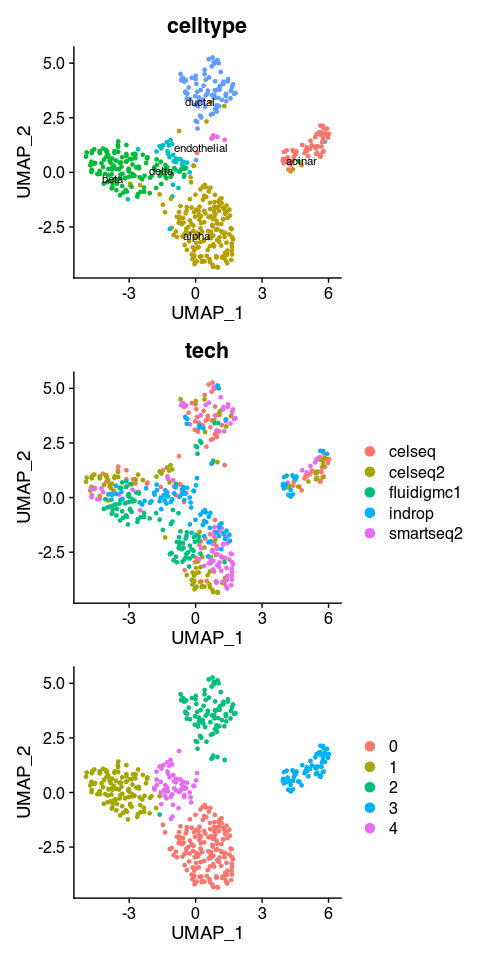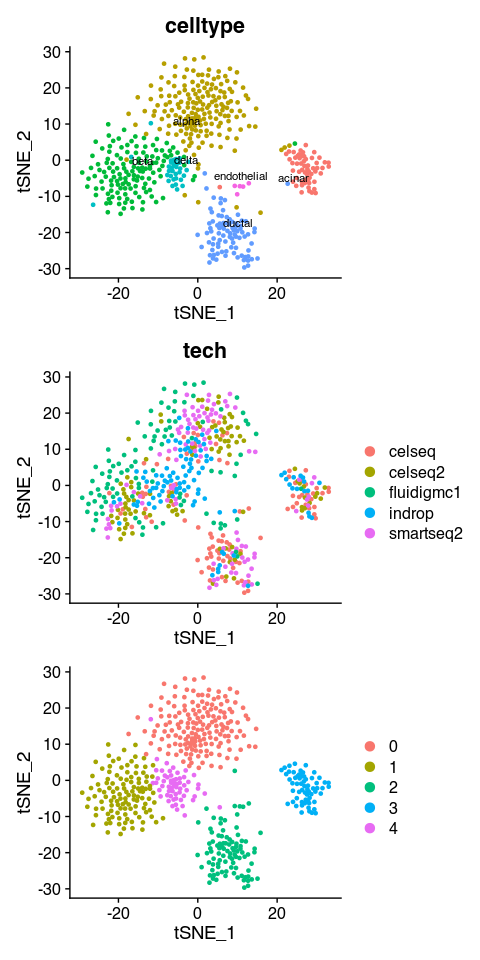# bbknnR Tutorial

## Setup library and data

library(bbknnR)
#> Registered S3 method overwritten by 'spatstat.core':
#>   method          from
#>   formula.glmmPQL MASS
library(Seurat)
#> Attaching SeuratObject
library(dplyr)
#>
#> Attaching package: 'dplyr'
#> The following objects are masked from 'package:stats':
#>
#>     filter, lag
#> The following objects are masked from 'package:base':
#>
#>     intersect, setdiff, setequal, union
library(patchwork)
data("panc8_small")

## Run BBKNN

Note that RunBBKNN() also compute t-SNE and UMAP by default.

panc8_small <- RunBBKNN(panc8_small, batch_key = "tech")
#> Running BBKNN using RcppAnnoy...
#> BBKNN finish!
#> Running UMAP...
#> Running tSNE...
#> All done!

## Find Clusters using bbknn graph

panc8_small <- FindClusters(panc8_small, graph.name = "bbknn")
#> Modularity Optimizer version 1.3.0 by Ludo Waltman and Nees Jan van Eck
#>
#> Number of nodes: 500
#> Number of edges: 6418
#>
#> Running Louvain algorithm...
#> Maximum modularity in 10 random starts: 0.7235
#> Number of communities: 5
#> Elapsed time: 0 seconds

## Visualization

p1 <- DimPlot(panc8_small, reduction = "umap", group.by = "celltype", label = TRUE,
label.size = 3 , repel = TRUE) + NoLegend()
p2 <- DimPlot(panc8_small, reduction = "umap", group.by = "tech")
p3 <- DimPlot(panc8_small, reduction = "umap")

wrap_plots(list(p1, p2, p3), ncol = 1)p1 <- DimPlot(panc8_small, reduction = "tsne", group.by = "celltype", label = TRUE,
label.size = 3 , repel = TRUE) + NoLegend()
p2 <- DimPlot(panc8_small, reduction = "tsne", group.by = "tech")
p3 <- DimPlot(panc8_small, reduction = "tsne")

wrap_plots(list(p1, p2, p3), ncol = 1)